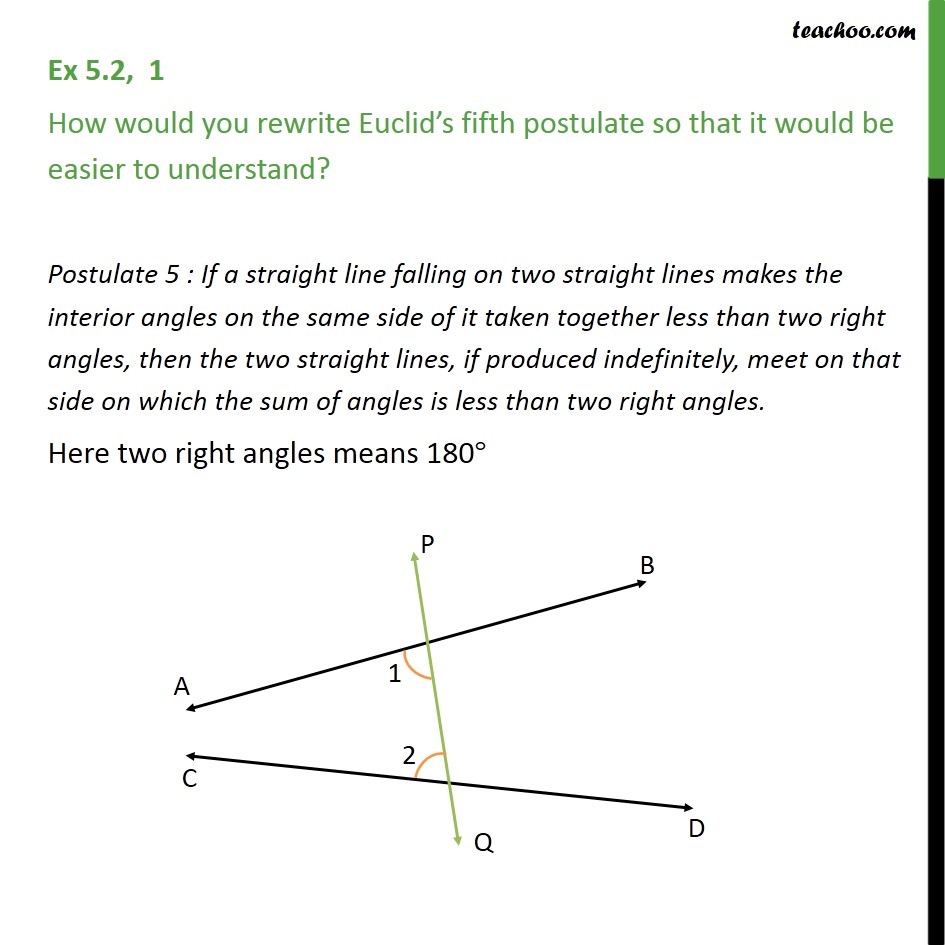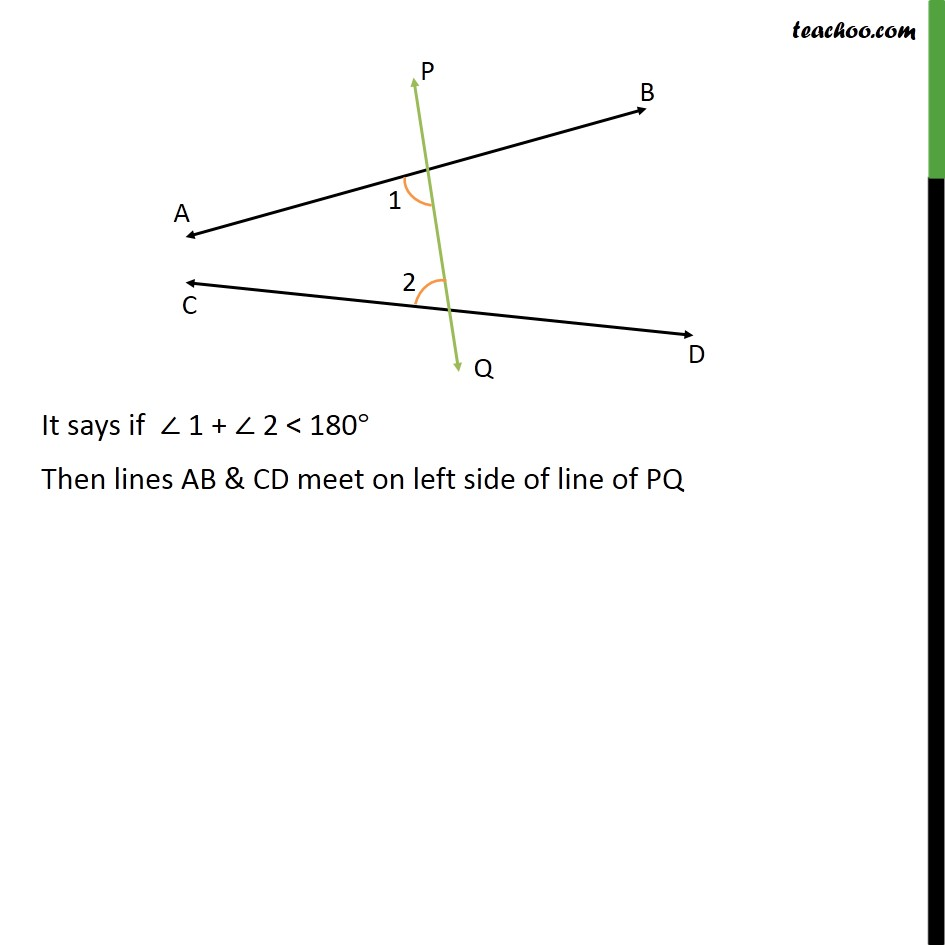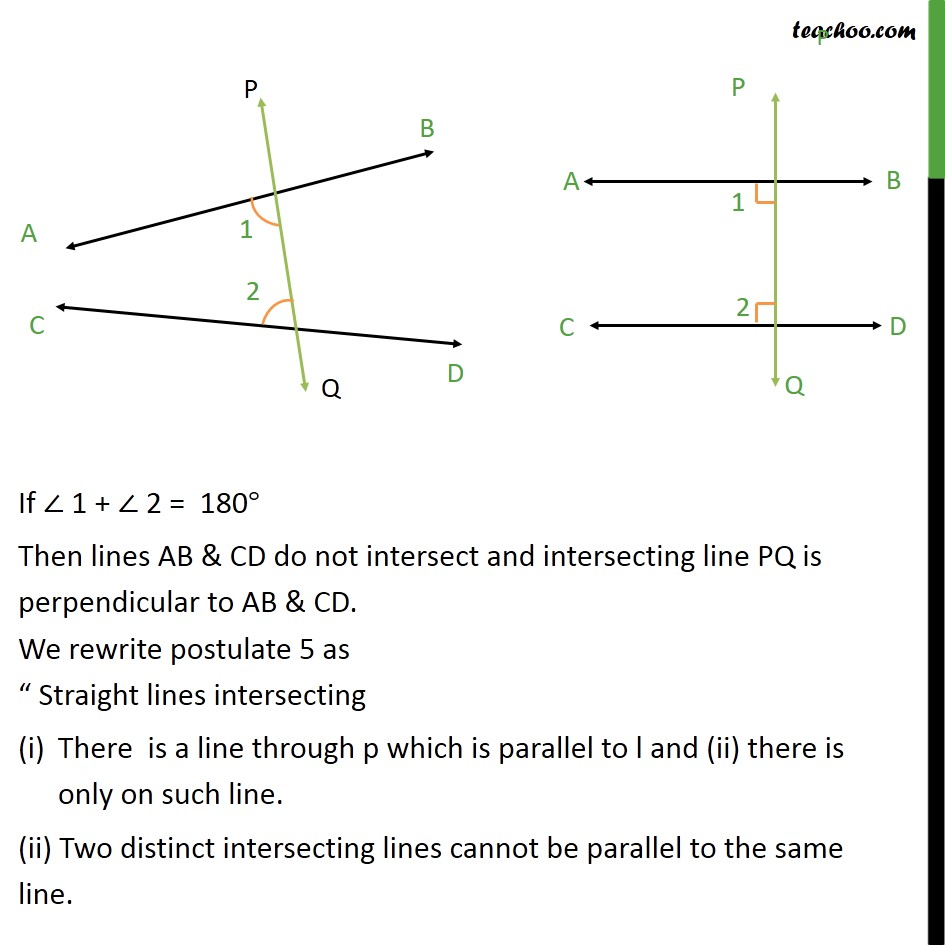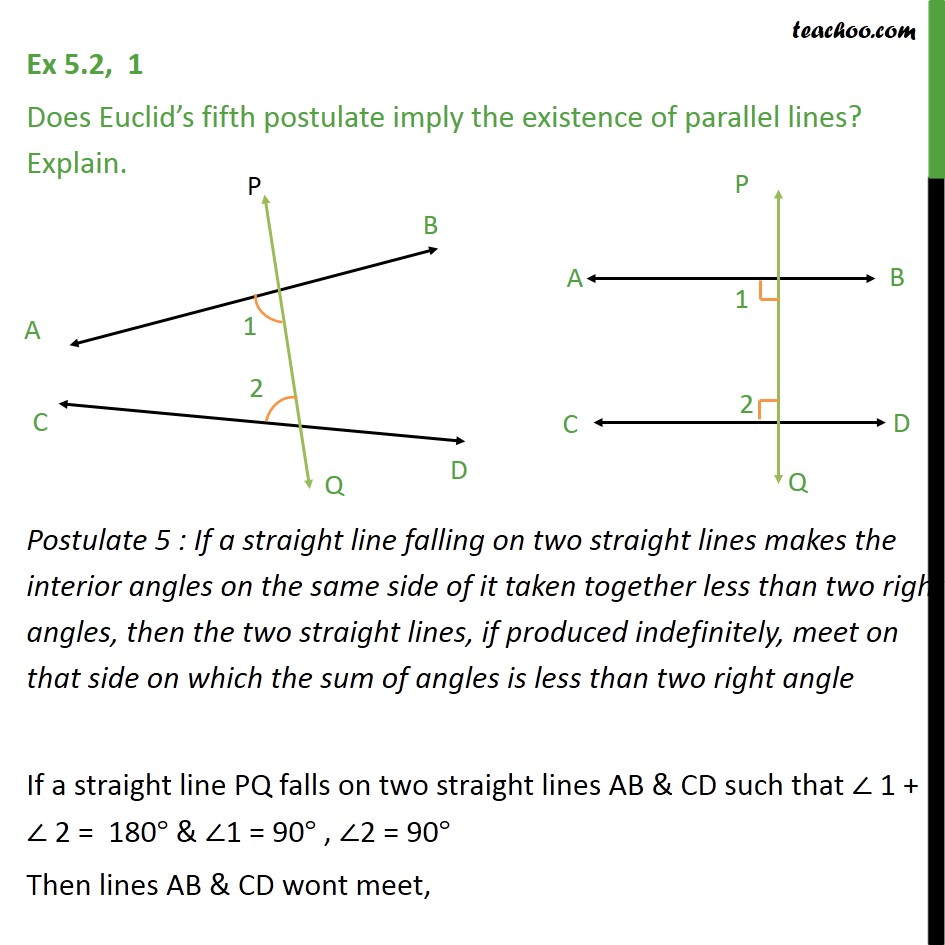Euclid's 5th Postulate

Chapter 5 Class 9 Introduction to Euclid's Geometry
Serial order wiseLearn in your speed, with individual attention - Teachoo Maths 1-on-1 Class

### Transcript

Question 1 How would you rewrite Euclid s fifth postulate so that it would be easier to understand? Postulate 5 : If a straight line falling on two straight lines makes the interior angles on the same side of it taken together less than two right angles, then the two straight lines, if produced indefinitely, meet on that side on which the sum of angles is less than two right angles. Here two right angles means 180 It says if 1 + 2 < 180 Then lines AB & CD meet on left side of line of PQ If 1 + 2 = 180 Then lines AB & CD do not intersect and intersecting line PQ is perpendicular to AB & CD. We rewrite postulate 5 as Straight lines intersecting There is a line through p which is parallel to l and (ii) there is only on such line. (ii) Two distinct intersecting lines cannot be parallel to the same line. Question 1 Does Euclid s fifth postulate imply the existence of parallel lines? Explain. Postulate 5 : If a straight line falling on two straight lines makes the interior angles on the same side of it taken together less than two right angles, then the two straight lines, if produced indefinitely, meet on that side on which the sum of angles is less than two right angle If a straight line PQ falls on two straight lines AB & CD such that 1 + 2 = 180 & 1 = 90 , 2 = 90 Then lines AB & CD wont meet, So, lines AB & CD are parallel.